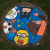Meta Learning，元学习，也叫 Learning to Learn（学会学习）。是继Reinforcement Learning（增强学习）之后又一个重要的研究分支。0+0+0+0+0+0+0+0+0+0+0+0+0+0+0+0+0+0+0+0+0+0+0+0+0+0+0+0+0+0+0+0+0+0+0+0+0+0+0+0+0+0+0+0+0+
Top